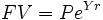# Interest

Interest is the cost of the use of money over time.

The interest rate is the percentage fee received or paid by individuals or organizations when they lend or borrow money. The formula for calculating interest in Principle x Rate x Time, or I=prt. Principle is the amount borrowed or still outstanding, Rate is the interest rate of the loan expressed as an annual amount and time is normally a year, or fraction thereof.

Interest rates have three main components:

Any interest rate must be at least the rate of inflation, or the money will be worth less when it is repaid than when it was lent.

Borrowers who are perceived to be a higher risk will be charged a higher interest rate. This allows the lender to recoup his losses from the higher risk borrowers who default via the excess received from those who don't.

The final component is the true cost of money. This is more theoretical then practical. However, risk free debt instruments during periods of no inflation often have a rate of 3%.

There are many different interest rates, but the benchmark interest rate—or overnight target rate—is set by the Central Bank of a nation typically. A change or expected change in this rate will lead to changes in other interest rates.

Interest is typically paid by banking institutions to customers who hold funds on long term deposit, as is done in a savings account or certificate of deposit. The reason the banks pay interest to depositors is that they are able to use these deposited funds in order to fund loans. One of the primary means by which banks generate revenue is in the differential between interest collected on loans and interest paid to depositors.

## Simple interest

The most basic way of expressing an interest rate is as a simple interest rate. In the case of a simple interest rate, a single rate is applied to the entire principle amount for the life of the loan. For example, a borrower takes a loan of $10,000 and agrees to a 5% simple interest rate. Over the life of the loan, the borrower will pay$10,500 back to the lender ($10,000 principle +$500 interest). Modern banks do not use simple interest in calculating interest rates for loans or savings.

## Compound interest

Modern banks use the concept of compound interest to calculate interest rates for loans and savings accounts. Compound interest works by taking the life period of the loan and dividing it into a number of periods (for example, dividing the year into 12 months) and then dividing the interest rate by the number of periods and compounding (calculating the interest balance) at the end of each period. For example, a borrower takes a loan of $10,000 and agrees to a 5% interest rate compounded quarterly. If the loan is paid back after four quarters, the borrower will pay$10,509.45. Banks can compound as often as they choose whether it be annually, quarterly, monthly, or even daily. Theoretically banks could compound interest for a customer continuously, though this produces a diminishing return for the amount of work needed to calculate interest payments for all loans and deposits.

Compound interest calculations can be reduced to a simple formula:The Final Value (FV) is the Principle (P) multiplied by the value e to the power of the number of years of the loan (Y) multiplied by the interest rate (R).